Tip: Other languages are Google-Translated. You can visit the English version of this link.orRegisteror

## How to convert currency to words text in Excel?

For non-native speakers who use English as their second language, sometimes is hard to directly change the currency number to English words when the number is too long. In this article, you will learn how to easily convert currency to words text in Excel.

Convert currency to words with VBA code

Convert currency to words with Kutools for Excel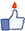Easily convert currency number to English word in Excel with Kutools for Excel

Click Kutools > Content > Spell Out Numbers. The Kutools for Excel's Spell Out Numbers utility can help you easily convert all currency numbers to English word in Excel. See below screenshot: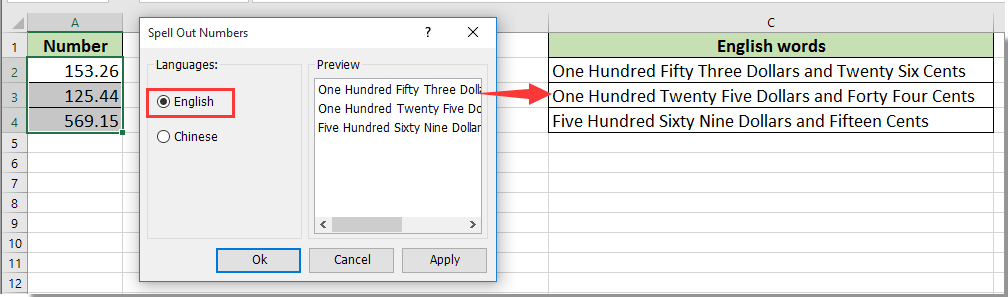####Convert currency to words with VBA code###### Save 50% of your time, and reduce thousands of mouse clicks for you every day!

With the below VBA code, you can convert currency number to English words.

1. Press Alt + F11 to open the Microsoft Visual Basic for Applications dialog box.

2. In the Microsoft Visual Basic for Applications dialog box, click Insert > Module. Then copy and paste the below code into the code window.

VBA code: Convert currency number to English Words

```Function NumberstoWords(ByVal pNumber)
Dim Dollars, Cents
arr = Array("", "", " Thousand ", " Million ", " Billion ", " Trillion ")
pNumber = Trim(Str(pNumber))
xDecimal = InStr(pNumber, ".")
If xDecimal > 0 Then
Cents = GetTens(Left(Mid(pNumber, xDecimal + 1) & "00", 2))
pNumber = Trim(Left(pNumber, xDecimal - 1))
End If
xIndex = 1
Do While pNumber <> ""
xHundred = ""
xValue = Right(pNumber, 3)
If Val(xValue) <> 0 Then
xValue = Right("000" & xValue, 3)
If Mid(xValue, 1, 1) <> "0" Then
xHundred = GetDigit(Mid(xValue, 1, 1)) & " Hundred "
End If
If Mid(xValue, 2, 1) <> "0" Then
xHundred = xHundred & GetTens(Mid(xValue, 2))
Else
xHundred = xHundred & GetDigit(Mid(xValue, 3))
End If
End If
If xHundred <> "" Then
Dollars = xHundred & arr(xIndex) & Dollars
End If
If Len(pNumber) > 3 Then
pNumber = Left(pNumber, Len(pNumber) - 3)
Else
pNumber = ""
End If
xIndex = xIndex + 1
Loop
Select Case Dollars
Case ""
Dollars = "No Dollars"
Case "One"
Dollars = "One Dollar"
Case Else
Dollars = Dollars & " Dollars"
End Select
Select Case Cents
Case ""
Cents = " and No Cents"
Case "One"
Cents = " and One Cent"
Case Else
Cents = " and " & Cents & " Cents"
End Select
NumberstoWords = Dollars & Cents
End Function
Function GetTens(pTens)
Dim Result As String
Result = ""
If Val(Left(pTens, 1)) = 1 Then
Select Case Val(pTens)
Case 10: Result = "Ten"
Case 11: Result = "Eleven"
Case 12: Result = "Twelve"
Case 13: Result = "Thirteen"
Case 14: Result = "Fourteen"
Case 15: Result = "Fifteen"
Case 16: Result = "Sixteen"
Case 17: Result = "Seventeen"
Case 18: Result = "Eighteen"
Case 19: Result = "Nineteen"
Case Else
End Select
Else
Select Case Val(Left(pTens, 1))
Case 2: Result = "Twenty "
Case 3: Result = "Thirty "
Case 4: Result = "Forty "
Case 5: Result = "Fifty "
Case 6: Result = "Sixty "
Case 7: Result = "Seventy "
Case 8: Result = "Eighty "
Case 9: Result = "Ninety "
Case Else
End Select
Result = Result & GetDigit(Right(pTens, 1))
End If
GetTens = Result
End Function
Function GetDigit(pDigit)
Select Case Val(pDigit)
Case 1: GetDigit = "One"
Case 2: GetDigit = "Two"
Case 3: GetDigit = "Three"
Case 4: GetDigit = "Four"
Case 5: GetDigit = "Five"
Case 6: GetDigit = "Six"
Case 7: GetDigit = "Seven"
Case 8: GetDigit = "Eight"
Case 9: GetDigit = "Nine"
Case Else: GetDigit = ""
End Select
End Function```

3. Press Alt + Q keys simultaneously to close the Microsoft Visual Basic for Applications dialog box.

4. Select a blank cell (B1) which is adjacent to the cell you want to convert to words, enter the formula =NumberstoWords(A1), and then press the Enter key.Note: A1 is the cell contains the currency number. You can change it as you need.

5. Select the cell B1, drag the Fill Handle down to get all English words of the currency numbers.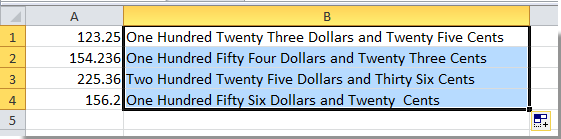####Convert currency to words with Kutools for Excel

This long VBA code seems complicated. Here I will introduce you a handy utility to easily solve this problem. With the Spell Out Numbers utility of Kutools for Excel, converting currency to words won’t be a problem anymore. Please do as follows.

 : with more than 300 handy Excel add-ins, free to try with no limitation in 60 days.

1. Select the cells with the currency numbers you want to convert.

2. Click Kutools > Content > Spell Out Numbers. See screenshot: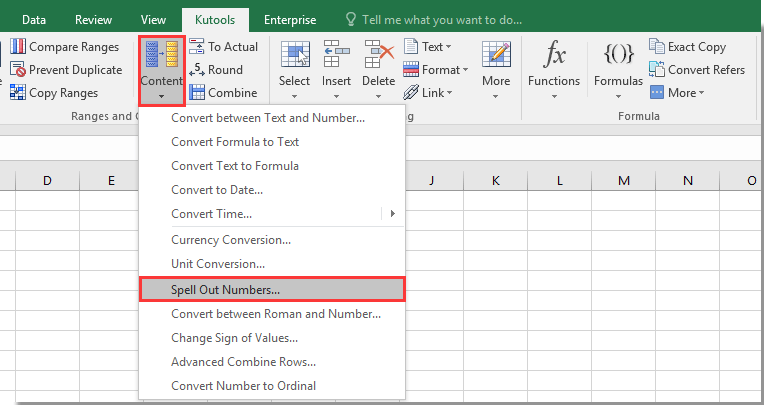3. In the Spell Out Numbers dialog box, select English option, and click the OK or Apply button.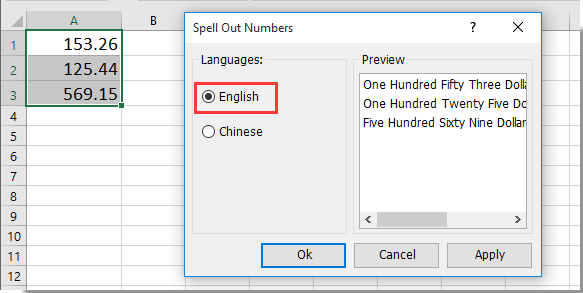Now the selected currency numbers are converted to English words immediately.You can

### Kutools for Excel Helps You Always Finish Work Ahead of Time, and Stand Out From Crowd

• More than 300 powerful advanced features, designed for 1500 work scenarios, increasing productivity by 70%, give you more time to take care of family and enjoy life.
• No longer need memorizing formulas and VBA codes, give your brain a rest from now on.
• Become an Excel expert in 3 minutes, Complicated and repeated operations can be done in seconds,
• Reduce thousands of keyboard & mouse operations every day, say goodbye to occupational diseases now.
• 110,000 highly effective people and 300+ world-renowned companies' choice.
• 60-day full features free trial. 60-day money back guarantees. 2 years of free upgrade and support.

### Brings Tabbed Browsing and Editing to Microsoft Office, Far More Powerful Than The Browser's Tabs

• Office Tab is designed for Word, Excel, PowerPoint and Other Office Applications: Publisher, Access, Visio and Project.
• Open and create multiple documents in new tabs of the same window, rather than in new windows.
• Increases your productivity by 50%, and reduces hundreds of mouse clicks for you every day!Say something here...
symbols left.